Trial ends in
JoVE Science Education
General Chemistry

A subscription to JoVE is required to view this content.
You will only be able to see the first 20 seconds.

Determining the Empirical Formula
##### Science Education (General Chemistry)
Freezing-Point Depression to Determine an Unknown Compound
##### Science Education (General Chemistry)
Determining the Mass Percent Composition in an Aqueous Solution

# Determining the Empirical Formula

### Overview

Source: Laboratory of Dr. Neal Abrams - SUNY College of Environmental Science and Forestry

Determining the chemical formula of a compound is at the heart of what chemists do in the laboratory every day. Many tools are available to aid in this determination, but one of the simplest (and most accurate) is the determination of the empirical formula. Why is this useful? Because of the law of conservation of mass, any reaction can be followed gravimetrically, or by change in mass. The empirical formula provides the smallest whole-number ratio among elements (or compounds) within a molecular compound. In this experiment, gravimetric analysis will be used to determine the empirical formula of copper chloride hydrate, CuxCly·nH2O.

### Principles

Hydrates are chemical compounds that have water molecules attached (but not covalently bonded) to the compound. Formulas that are hydrated are symbolized by a dot ("·") between the compound and the water molecule. Hydrates easily lose water molecules upon heating, leaving behind the anhydrous (without water) compound. In this case, it would be copper chloride, CuxCly. The difference in mass between the anhydrous and hydrated forms of the salt corresponds to the mass (and moles) of water in the chemical compound CuxCly·nH2O. The anhydrous copper chloride is then dissolved in water, and copper is removed through a redox reaction with aluminum to form solid copper. The difference in mass between the total copper chloride hydrate and the sum of the reduced copper metal and water molecules corresponds to the mass of chloride in the sample. The mass of each component (Cu, Cl, H2O) is converted to moles, whereby the law of multiple proportions allows the use of a ratio to determine the empirical formula of the compound. The true chemical formula of the compound cannot be determined without knowing its molecular mass, but the ratio will always remain the same.

Subscription Required. Please recommend JoVE to your librarian.

### Procedure

1. Dehydrating the Hydrate

1. Accurately weigh a sample of copper chloride hydrate and place it into a pre-dried and tared crucible. It is important that the crucible is dried above 120 °C to drive off any adsorbed moisture. Typically, 1–2 g of compound will suffice.
2. Heat the sample using a Bunsen burner or other flame source until it changes color from greenish-blue to a reddish-brown (Figure 1). This color change is indicative of the anhydrous form of copper chloride. The cover can stay on the crucible to prevent splattering, but should be opened slightly to allow water vapor to escape.
1. Stir the sample to be sure the water is driven off the entire sample and the color is constant throughout.
2. As an alternative, the sample can be placed into a drying oven above 110 °C.
3. Cool the sample in a desiccator. This prevents water from rehydrating the sample.
4. Measure the mass of the anhydrous sample. The difference corresponds to the water from the hydrate that was lost upon heating.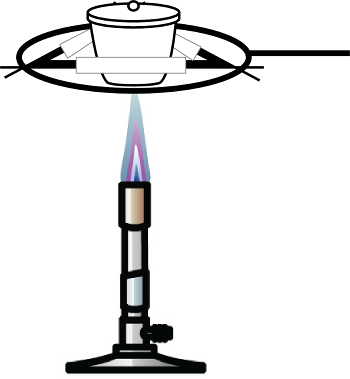Figure 1. Bunsen burner with ceramic crucible.

2. Isolating Copper

1. Transfer the sample to a 100 mL beaker and dissolve the sample in 50 mL of deionized water. The solution should turn blue once again, typically more blue than the hydrated solid.
2. Add a small quantity (~0.20 g) of aluminum metal to the beaker. This will cause the copper to reduce to a reddish metal, and the aluminum will oxidize to colorless Al3+. The blue color of the solution should disappear as the Cu2+ ions form Cu0. After 30 min, add additional small pieces of aluminum to ensure all of the copper is reduced to solid copper.
1. The solution now contains Al3+ ions, solid copper, and a small amount of solid aluminum.
3. Dissolve any excess aluminum by adding ~5 mL of 6 M HCl. Aluminum is amphoteric, meaning it can react and dissolve in the presence of an acid or a base.
4. Vacuum filter the colorless solution in a Büchner funnel containing a pre-weighed piece of filter paper. Rinse with absolute ethanol. Air dry (not oven dry) the sample to prevent the formation of copper (II) oxide.
5. Measure the mass of the copper solid to determine the mass of the chloride ion by difference.

3. Calculations

1. Determine the mass of the chloride ion by difference: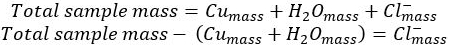2. Use the molar mass of each component of the compound to determine the moles of each component.
3. Divide the moles of each component by the moles of the smallest component to give the smallest whole-number ratio of components, also known as the empirical formula of the compound.

Determining the chemical formula of a compound is a fundamental aspect of a chemist's occupation.

In a chemical formula, element symbols and numerical subscripts describe the types and number of atoms present in a molecule. The empirical formula is a simple type of chemical formula, which provides the smallest whole-number ratio among elements within a molecular compound. Because of the law of conservation of mass, the empirical formula is often found using elemental composition or mass percentage.

This video will introduce the empirical formula and demonstrate how it can be calculated using a simple experiment in the laboratory.

The empirical formula is the simplest type of chemical formula, as it shows the relative number of atoms of each element in a given compound. For example, in hydrogen peroxide, there is one part by mass of hydrogen for every 16 parts by mass of oxygen. Therefore for every hydrogen atom, there is one oxygen atom, and the empirical formula is H-O. Many different molecules may have the same empirical formula.

The molecular formula is related to the empirical formula, and represents the actual number of atoms of each type in a compound. For example, the molecular formula of hydrogen peroxide is H2O2, as each molecule has two hydrogen atoms and two oxygen atoms. A structural formula shows the number of each type of atom, and the bonds between them. Single lines represent a chemical bond. For example, for hydrogen peroxide the structural formula looks like this: H-O-O-H.

Formulas with a dot between the compound and water describe hydrates. Hydrates are chemical compounds that have water molecules attached, but not covalently bonded. Hydrates easily lose their water molecules upon heating and become “anhydrous,” or “without water.” Hydrates and anhydrous compounds have unique physical properties, as the molecules organize differently.

Now that the basic principles of the empirical formula have been explained, lets confirm the empirical formula of a copper chloride hydrate in the laboratory.

To begin the procedure, dry the crucible above 120 °C to drive off any adsorbed moisture, and accurately determine its weight.

Weigh a sample of a copper chloride hydrate, and place it into the crucible.

Next, heat the sample in the crucible using a heat source, such as a Bunsen burner. Place the cover on the crucible to help prevent splattering, but keep it open slightly to allow water vapor to escape.

Heat the sample until it has changed from a blue-green color to a red-brown color. This color change is indicative of the anhydrous form of copper chloride. Stir to make sure that the water has been driven off the sample, and the color is consistent throughout.

Next, cool the sample in a desiccator, to prevent rehydration.

Accurately measure the mass of the anhydrous sample. The difference corresponds to the waters of hydration that were lost upon heating.

Transfer the dried sample into a 250 mL beaker, and dissolve it in 150 mL deionized water. The solution should turn blue again, as the copper chloride is rehydrated.

Add a small piece of aluminum wire to the beaker. The blue copper two plus will reduce to a reddish copper zero on the surface of the wire, while the aluminum will oxidize to colorless aluminum three plus. The blue color of the solution will disappear during the reaction.

After about 30 min, use additional aluminum to ensure that all of the copper has reduced to a solid copper metal.

Next, add about 10 mL of 6 M hydrochloric acid to dissolve the aluminum wire.

Using a Büchner funnel and pre-weighed filter paper, vacuum filter the colorless solution. Rinse the sample with absolute, or pure, ethanol. Allow the sample to air-dry.

Finally, measure the mass of the copper solid.

To determine the empirical formula of copper chloride hydrate, first calculate the mass of each component. The mass of water is determined by subtracting the weight of the dried copper chloride from the weight of the copper chloride hydrate. The mass of copper was found experimentally. Finally, the mass of chloride is found by subtracting the mass of copper and water from the total mass of the sample.

To determine the smallest whole-number ratio of components in the compound, convert the mass of each component to moles using the molar mass. Then divide each component by the smallest number of moles in the sample (copper in this case). The smallest whole-number ratio yields the formula of CuCl2·2H2O.

The determination and knowledge of the empirical formula of a compound is important in many areas of chemistry and research.

Forensic chemistry is the application of chemistry in a legal setting. For example, unknown compounds, such as drugs and poisons, are often found at crime scenes. Forensic chemists use a wide range of methods to identify the unknown substance.

Often, the next step in identifying an unknown substance is to use the empirical formula to determine the molecular formula. A mass spectrometer is frequently used to aid in this step, as the mass spectrometer separates components by their mass-to-charge ratio. Thus, the mass of the molecule can then be used to determine the molecular formula.

You've just watched JoVE's introduction to the empirical formula. You should now understand what the empirical formula of a substance is, how it differs from the molecular formula, and how to determine it in the laboratory.

Thanks for watching!

Subscription Required. Please recommend JoVE to your librarian.

### Results

1. Experiment
1. Heat 1.25 g of copper chloride hydrate in a crucible. After heating and then cooling, the final mass is 0.986 g of copper chloride, CuxCly.
2. Dissolve the CuxCly sample in 50 mL of deionized water and add 0.2 g of fine aluminum mesh to the beaker.
3. After reacting and dissolving the excess aluminum, 0.198 g of dried copper metal is recovered.
4. Subtract the mass of both copper and water from the initial copper chloride hydrate to yield the mass of chloride ion in the sample: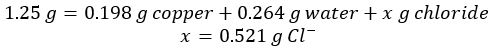2. Data
1. To determine the smallest whole-number ratio of components in the compound, convert the mass of each component to moles and then divide each by the smallest number of moles in the sample (copper in this case):
 Component Mass (g) Molar mass (g/mol) Moles Ratio Calculated whole-number ratio Copper 0.479 63.55 7.53 x 10-31 Chloride 0.533 35.45 1.50 x 10-2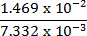1.99 ≈ 2 Water 0.273 18.01 1.51 x 10-2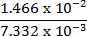2.01 ≈ 2

Table 1. Experimental results.

1. The resulting smallest whole-number ratio yields a formula of CuCl2·2H2O.
1. In the event the final ratio yields decimal values, the whole formula would be multiplied by a constant to give whole-number values. Common fractional values are 0.25, 0.333, 0.50, 0.667, and 0.75. For example, if a smallest whole-number ratio yielded the formula yielded C7H9NO2.5, the entire formula would be multiplied by 2 to give the empirical formula C14H18N2O5.
2. A molecular formula cannot be determined from the empirical formula without knowing the molecular mass of the compound. The reason for this is demonstrated in the example below:
 Name Molecular Formula Empirical Formula Acetic acid CH3COOH CH2O Formaldehyde CH2O CH2O Glucose C6H12O6 CH2O

Table 2. Example of a common empirical formula.

All three compounds have the same empirical formula, but very different molecular formulas.

Subscription Required. Please recommend JoVE to your librarian.

### Applications and Summary

In one example, suppose an unknown biomolecule containing only C, H, and O is found to act well as a new fuel. One way to determine the formula of the fuel would be to combust it in air and analyze the products:

CxHyOz + O2 → mCO2 + nH2O

While O2 is in excess, we would know all the carbon in CO2 originated from the biomolecule and all the hydrogen would be present in H2O. The difference between that total mass and the mass of initial sample would be the mass of oxygen in the molecule. We could then convert to moles and determine the empirical formula.

In another example, a hydrate sample of MgxCly·nH2O is given. The mass of the water molecules would again be easily determined by heating. Using some solubility rules, chloride is then precipitated with silver ion, Ag+, to form AgCl(s). Once the mass of AgCl(s) is found, the moles of Cl- are determined using the molar mass of AgCl(s) and then converted to grams of Cl-. This would allow us to determine the mass of Mg in the sample followed by the empirical formula.

Determining an empirical formula is at the center of identifying the formula of the actual molecule. From pharmaceuticals to forensics, determination of a molecular formula is key to identifying an unknown compound, which means taking the empirical formula to the next step. Typically, the determination of an empirical formula is coupled with elemental analysis to obtain elemental weight percent information. From these data, the molar ratios are calculated and the empirical formula is determined. We can determine the mass of molecule using another analytical tool, like a mass spectrometer. Then, the ratio between the molecular mass and empirical mass is calculated to determine the true molecular formula.

Subscription Required. Please recommend JoVE to your librarian.

### Get cutting-edge science videos from JoVE sent straight to your inbox every month.X